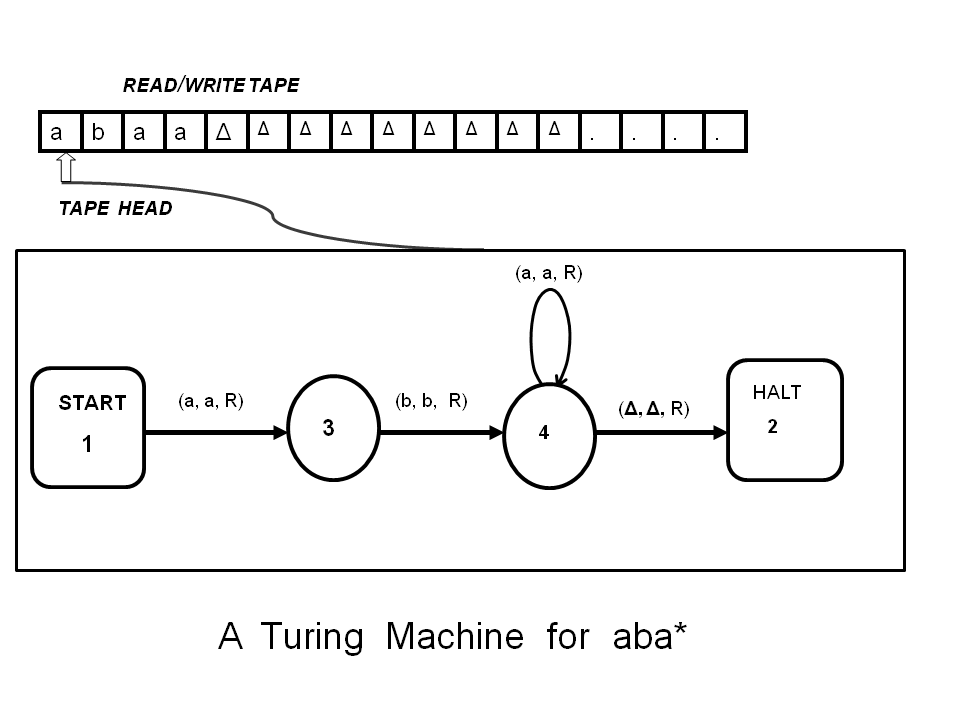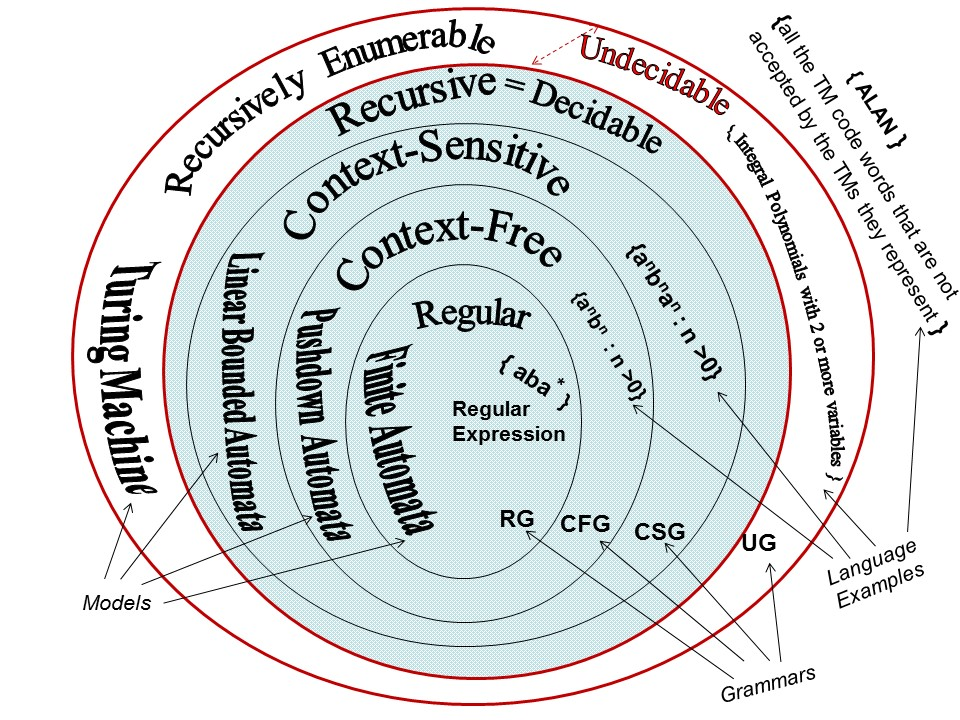### Learning Turing Machines in Asynchronous Mode

Consider the Turing Machine (TM) presented below. Its START state is marked by 1; the execution of the machine begins from the START state. The out going arrow from the START state to state 3 is a transition which is marked by the triplet (a, a, R). This triplet means read an a from the current cell of the READ/WRITE tape, write an a on the same cell, and move right one cell on the tape. Please use START_ANIMATION button in order to get started with learning in the asynchronous mode.Turing Machines (TMs) define the most powerful class of automata for processing the most complex sets, namely, the recursively enumerable sets. A TM has a finite set of states with one START state and some HALT states and an infinitely long READ/WRITE tape devided into distinct cells. The input is initially placed on the tape starting from the left most cell. The TAPE HEAD initially points to the leftmost cell. A TM for     aba*    is shown in action with an input string "abaa" . Press the START_ANIMATION button for invoking the animation. The machine starts execution from the start state. The moving short arrow shows the execution path and the colored input symbol on the tape shows the current symbol being processed.   (a, a, R)   on a transition arrow means read an a from the current tape-cell, write an a to the same cell, and move Right on the tape. For more on TMs

Figure 1. A Turing Machine (TM) for aba*

Any Turing Machine (TM ) can be represented in a table and then the table can be encoded into a string. The above TM is represented in Table 1 as shown below:

```

1          3          a        a         R
3          4          b        b         R
4          4          a        a         R
4          2          ∆        ∆         R

Table 1  The TM for aba* is represented in tabular form
```

In Table 1, each row represents a transition. The interpretation of the topmost row is that from state 1 to state 3, there is a transition that reads the symbol a, writes the symbol a, and moves Right . Using a method suggested by Cohen, D. (1997)[ Introduction to Computer Theory (2nd ed.), New York: John Wiley ], each transition of the TM (that is, each row of Table 1) can be coded into a string of a's and b's as shown in Table 2. In the first transition of the TM, (that is, in the top row of Table 1), state 1 is represented by one a, state 3 is represent by three a's after one b, which serves as a separator (SEP in Table 2). After that, one b serves as a separator. Then, aa stands for reading an a , and the next aa stands for writing an a, and then the 11th symbol, b, stands for moving Right.

```

FROM  SEP   TO   SEP  READ     WRITE      MOVE   Concatenated Code

1          3          a        a         R
a    b    aaa   b     aa       aa        b       abaaabaaaab

3          4          b        b         R

aaa   b   aaaa   b     ab       ab        b       aaabaaaabababb

4          4          a        a         R
aaaa   b   aaaa   b     aa       aa        b       aaaabaaaabaaaab

4          2          ∆        ∆         R
aaaa   b    aa    b     ba       ba        b       aaaabaabbabab

Table 2.  Each Row of  Table 1 is coded in a's and b's in red color immediately below that row

```

In Table 2, SEP stands for separator. For READ & WRITE, an a is coded as aa, a b is coded as ab, and a ∆ is coded as ba. For MOVE, R (right), is coded as b and L (left) is coded as a. Please note that in each coded row, after the 2nd b (which is a separator), there are exactly five coded symbols. The concatenated coded string for the entire TM is:
abaaabaaaabaaabaaaabababbaaaabaaaabaaaabaaaabaabbabab

Following Cohen (1997), the first eleven symbols (abaaabaaaab) of the code represent the first transition of the TM, that is, the top row of Table 2. State 1 is represented by one a, state 3 is represent by three a's after one b, which serves as a separator. After that, one b serves as a separator (SEP). Then, aa stands for reading an a , and the next aa stands for writing an a, and then the 11th symbol, b, stands for moving Right. Similarly, each transition is translated into a string. Coded TMs are used in proving important theorems. A TM can be run on its own code to check if it accepts its own code. Some TMs accepts their own code others do not accept their own code. If we run the TM of Figure 1 on its code obtained from Table 2 then it does not accept its own code. Consider the following language: ALAN = { all the code words that are not accepted the TMs they represent }. ALAN is not computable because if we assume that there is a TM, T, that accepts ALAN then it generates contradiction. ( For a proof, click here).

Following Cohen (1997), we define TMs as follows.
A TM is composed of six components:
1. An alphabet, Σ, which is a finite non-empty set of symbols from which input is built.
2. A READ/WRITE TAPE, divided into a sequence of numbered cells; each of the cells contains one character or a blank, Δ. The input is presented to the machine one letter per cell beginning in the leftmost cell. The rest of the tape is initially filled with blanks.
3. A TAPE HEAD points to the current letter being read from the READ/WRITE TAPE. It can in one step read the contents of the READ/WRITE TAPE, write a symbol on the tape and move left or right one cell.
4. An alphabet, Γ, of symbols for the READ/WRITE TAPE. This can include symbols of Σ.
5. A finite set of states including one start state from which execution of the machine begins and some (may be none) HALT states that cause execution to terminate when entered.
6. A set of transitions from state to state where each transition has three elements:

The first element of the triplet is a letter read by the TAPE HEAD of the machine from the current cell. The second element is a letter written on the tape on the same cell where the first element was read from. The third element, Move, tells the TAPE HEAD whether to move one cell right, R, or one cell left, L. The HALT state cannot have any outgoing transition. To terminate execution on certain input successfully, the machine must be led to a HALT state. The input is then said to be accepted by the machine.

The TM presented in Figure 1 (at the top of this page) accepts every string in the regular expression, aba*. The language denoted by the regular expression aba* is a set of strings which can be written as: L1 = aba* = {ab, aba, abaa, abaaa, abaaaa, abaaaaa, . . . }. Can you think about a set for which no TM can be constructed? That set would be an un-computable set.

The languages that TMs can recognize are called Turing recognizable languages or recursively enumerable languages; these languages can be classified into a number of types which are shown to be properly included as subsets of the recursively enumerable languages in Figure 2 (following Cohen 1997).Figure 2. Hierarchy of Languages and Automata

Finite Automata can recognize regular languages shown in the innermost circle of Figure 2. However, Finite Automata cannot recognize non-regular Context-Free languages. Pushdown Automata can recognize Context-Free languages and regular languages because regular languages are properly included in Context-Free languages. Similarly recursively enumerable sets (or languages) properly include all recursive sets including Context-Sensitive sets (or languages), Context-Free sets (or languages) and regular languages. However the term undecidable languages (or sets) is used in a special way to exclude recursive or decidable languages. There are sets which are undecidable. Given a candidate input if you run the appropriate TM (or algorithm) on that input then the TM may loop for ever without halting and accepting or rejecting the input. The set of two variable integral polynomials is undecidable. You can run the following program for getting an intuitive idea about undecidability of the set of two variable integral polynomials.

The set of two variable integral polynomials is undecidable
This program returns the value of x & y if the input is a polynomial with integer values for x & y

Please enter integers in the input fields and press the "DECIDE THE INPUT POLYNOMIAL" button
x3y2 + x2y + xy - = 0

[ You can try your example equations. If you try   2x3y2 + 4x2y + 2xy - 500 = 0, the program will return 2 as the value of x and 5 as the value of y. If you try   2x3y2 + 4x2y + 2xy - 1032 = 0, the program will return 3 as the value of x and 4 as the value of y. You need to enter only integers as coefficients in the input fields, the rest of the equation is fixed. There are no lower & upper bounds for integral polynomials with 2 or more variables; therefore, the program may run for ever on most examples. One of the undecidable integral polynomial is:     2x3y2 + 4x2y + 2xy - 5 = 0 . Matiyasevich's theorem proves that multivariable integral polynomials are undecidable. ]

A set (or language), L, is recognizable (or recursively enumerable) but undecidable if there exists a TM, M, such that (1) if the input string w belongs to L and M is run with input w, then M halts and accepts it (with acceptable solutions). (2) If the input string w does not belong to L and M is run with the input string w, then M does NOT halt (that is, it runs for ever). L is undecidable if there is no TM, M, such that M halts on every input either rejecting it or accepting it.   Please send comments to:   Pradip Peter Dey ( pdey@nu.edu)